You are using an outdated browser. Please upgrade your browser to improve your experience.Equation Solver 4-in-1 is the ultimate app that helps you solve linear equations in one variable, quadratic equations with real and complex roots, cubic and quartic equations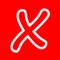Equation Solvers

by Intemodino Group s.r.o.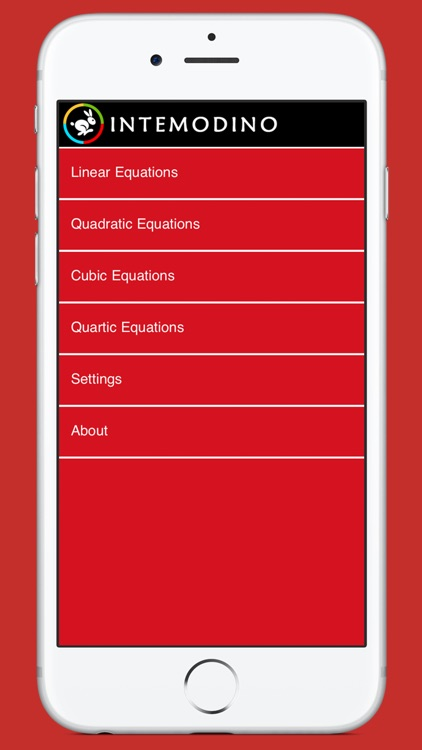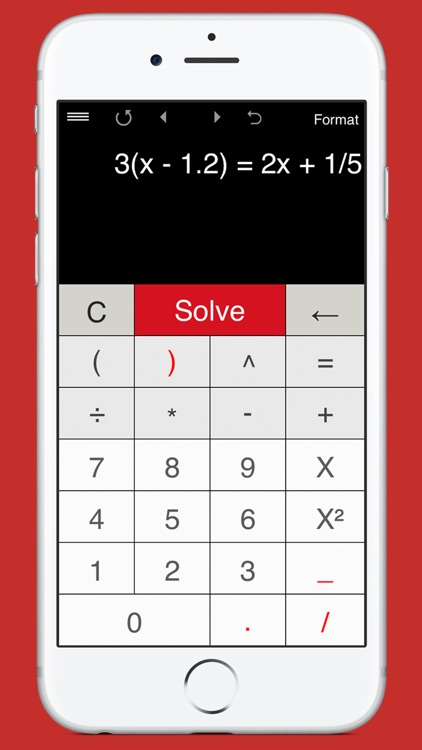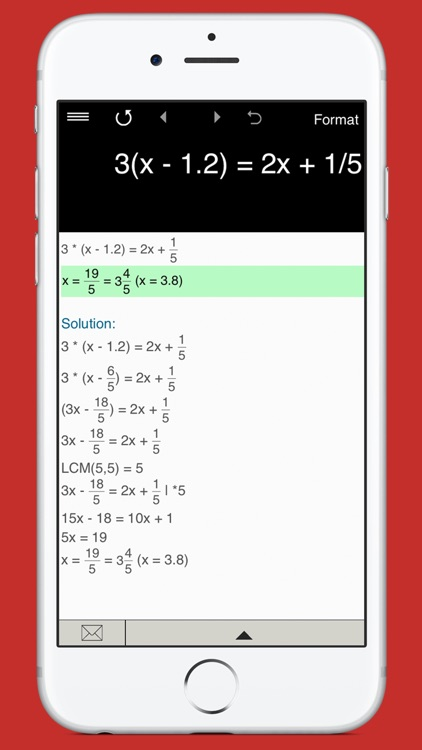What is it about?

Equation Solver 4-in-1 is the ultimate app that helps you solve linear equations in one variable, quadratic equations with real and complex roots, cubic and quartic equations. It's intuitive and easy to use. Just type in any equation you want to solve and the equation calculator will show you the result.App Details

Version
4.0.3
Rating
(18)
Size
26Mb
Genre
Education Utilities
Last updated
August 24, 2018
Release date
November 9, 2012

App Screenshots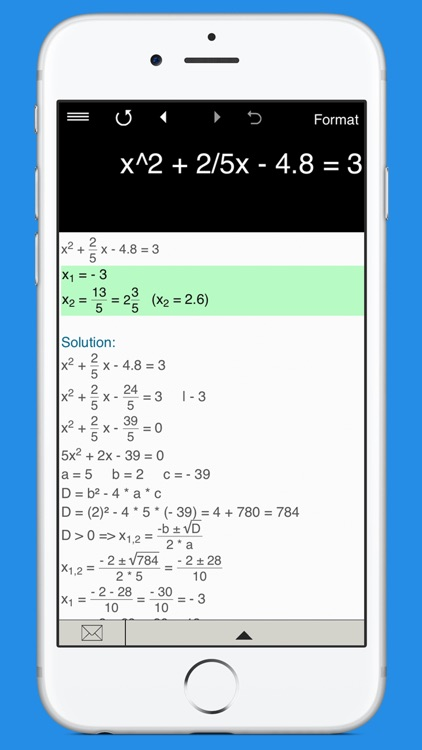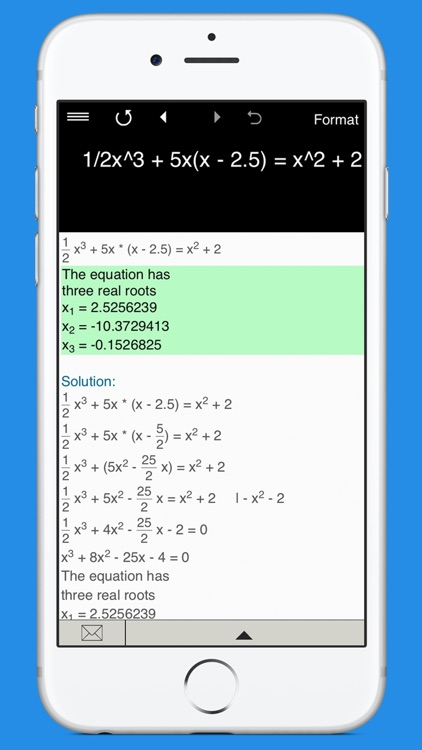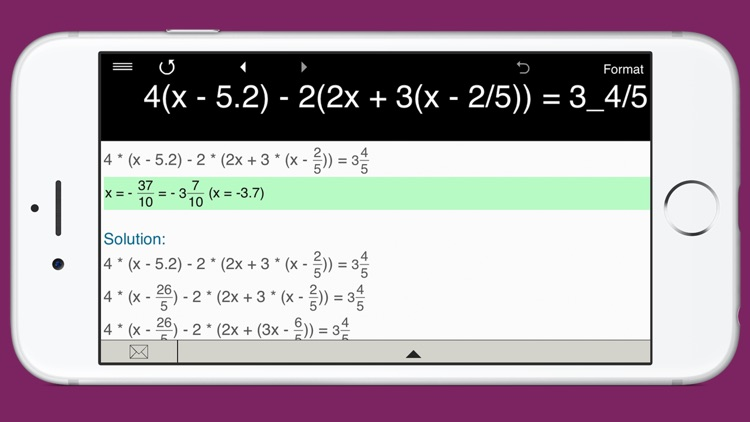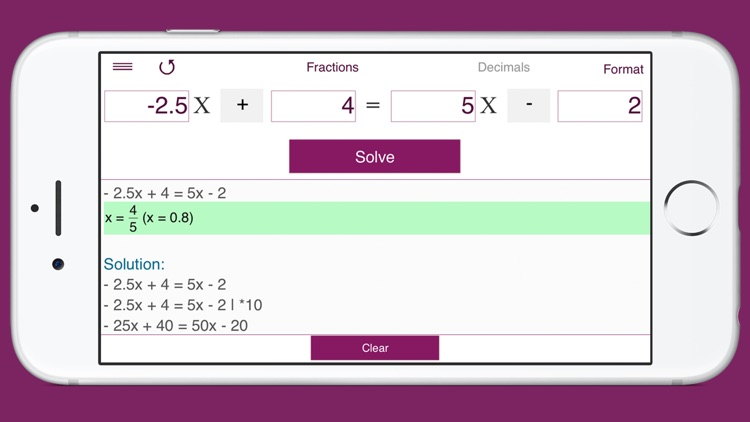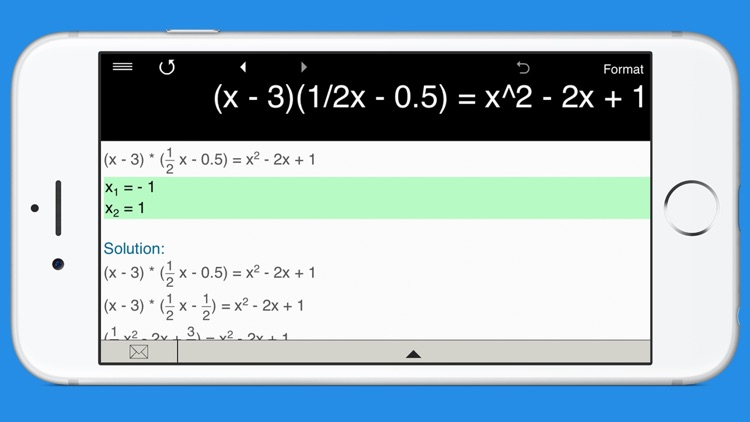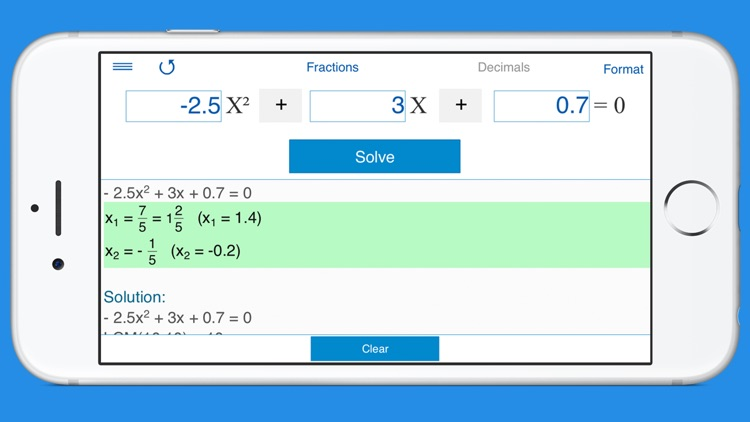App Store Description

Equation Solver 4-in-1 is the ultimate app that helps you solve linear equations in one variable, quadratic equations with real and complex roots, cubic and quartic equations. It's intuitive and easy to use. Just type in any equation you want to solve and the equation calculator will show you the result.

Everything you need to solve equations or check results!

Features:

• Linear Equation Solver.
• Quadratic Equation Solver.
• Cubic Equation Solver.
• Quartic Equation Solver.
• Solves equations with fractions and parentheses. Note: the app doesn’t yet support equations with a variable in the denominator.
• Supports integers, fractions and decimals, including negative values.
• Predefined formats.
• Detailed step-by-step instructions for linear equations and quadratic equations.
• Stores history with the possibility to recall previous equations.
• Back and forward buttons to check and recall recent equations.
• Sends results and history via email.

Disclaimer:
AppAdvice does not own this application and only provides images and links contained in the iTunes Search API, to help our users find the best apps to download. If you are the developer of this app and would like your information removed, please send a request to [email protected] and your information will be removed.